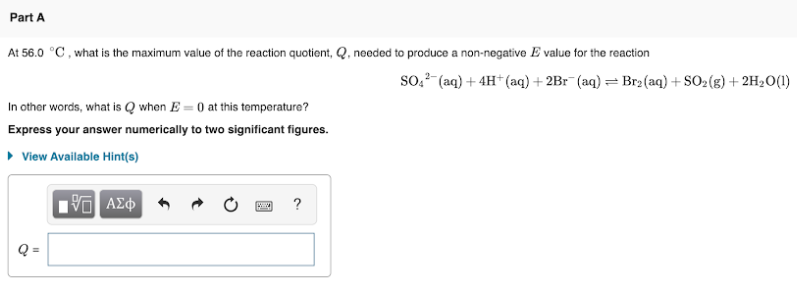# At 56.0 °C, what is the maximum value of the reaction quotient, Q, needed to produce a no-negative E value for the reaction SO4^2-(aq) + 4H^+(aq) + 2Br^-(aq) ⇌ Br2(aq) + SO2(g) + 2H2O(l) In other words, what is Q when E = 0 at this temperture? Express your answer numerically to two significant figures.